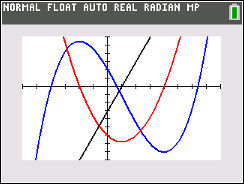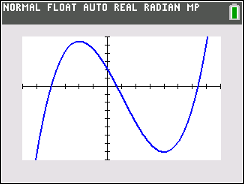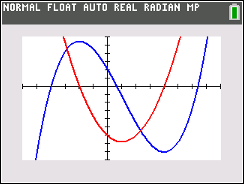••• ##### Device
• TI-83 Plus Family
• TI-84 Plus
• TI-84 Plus Silver Edition
•TI-84 Plus C Silver Edition
•TI-84 Plus CE

# Calculus: Graphing Relationships

by Texas Instruments#### Overview

In this activity, students explore information about a graph based on the first and second derivatives. They learn that a function's derivative is positive when the function increases and negative when the function decreases. They learn that the second derivative is positive when the graph is concave upward and negative when the graph is concave downward.

#### Key Steps

•Students will learn that a function’s derivative is positive when the function increases and negative when the function decreases.

•Students will learn that the second derivative is positive when the graph is concave upward and negative when the graph is concave downward.